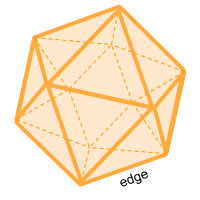Home » Polyhedra » Platonic Solids » Icosahedron

# Volume of an IcosahedronVolume =
5 × (3 + √5)/12
× Edge ³

## Volume calculator for an icosahedron

Enter the length of the edge of the icosahedron to get the volume automatically.

## Description, how many faces, edges and vertices are there in an icosahedron

The icosahedron, (icosa = 20 and hedron = Face) has 20 faces where each of these faces is an equilateral triangle, has 30 edges and 12 vertices. At each of the vertices, 5 edges meet.

## Examples of an icosahedron

We can find many objects that look like an icosahedron, examples of icosahedron shaped objects are: 20-sided dice that are used in some board games. Some viruses that affect health are icosahedron, one of them is the human papilloma virus.

Another famous object that could be considered an icosahedron are soccer balls, but they are actually truncated icosahedra, they have a cut on its surface creating 12 pentagons and 20 hexagons. Can you think of any other examples? leave a comment in the comment section at the bottom of the page.

## Formula for the volume of an icosahedron

To calculate the volume of a icosahedron you can calculate the volume of 20 equal triangular pyramids joint by their vertex. The difficulty is being able to calculate the height of these pyramids, but we can make use of the following formula, where we only need the value of an edge. You can also use the online calculator to calculate the volume of the icosahedron automatically.

Volume =
5 × (3 + √5)/12
× Edge ³

Share